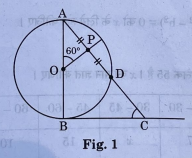# In Fig. 1, AB is diameter of a circle centered at 0. BC is tangent to the circle at B. If OP bisects the chord AD and ∠ACP = 60°, then find m∠C.

Class 10th Maths Term 2, Question - In Fig. 1, AB is diameter of a circle centered at 0. BC is tangent to the circle at B. If OP bisects the chord AD and ∠ACP = 60°, then find m∠C.## Maths Class 10 Term 2, Answer to the Question : In Fig. 1, AB is diameter of a circle centered at 0. BC is tangent to the circle at B. If OP bisects the chord AD and ∠ACP = 60°, then find m∠C.